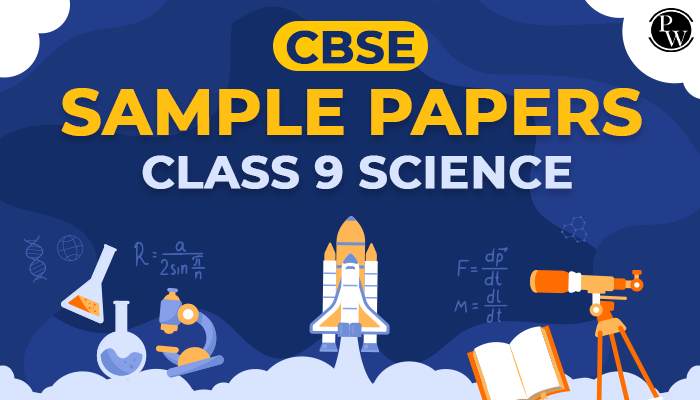# CBSE class 9 science sample paper

Sample papers for class 9 Science are prepared by the academic team of Physics Wallah. Each sample papers consist of a certain set of questions of all types like one marks, two marks, three marks and five marks questions with solutions. Few Sample papers for class 9 Science are uploaded without solutions too.

CBSE Sample Papers Class 9 Science from 1 to 5 consist of all types of questions and then after all sample papers consist of advance questions based on class 9 science and all are MCQ based for complete revision. Do solve NCERT Exercise with the help of NCERT Solutions for class 9 Science.## Find Below Sample papers for class 9 Science for reference

### Understand the pattern of class 9 Science

As per the CBSE guideline Class 9 Science Exam counts for total 100 marks. CBSE Class 9 science consist of two components one is theory paper of 80 marks and second is internal assessment of 20 marks. Internal marks will be divided among different activities such as Periodic Test (10 Marks), Practical Work(5 Marks), Portfolio(5 Marks). Find the right approach to use Sample paper. Do follow class 9 notes for reference.

### Sample papers for class 9 Maths design will be as per the following scheme

The class 9 Science test paper consist of 36 questions. All these questions will be divided into three sections as described below: The class 9 Maths test paper will comprise of a total of total 40 questions divided into four sections, such as A, B, C and D sections.

1. Section A will consist of 20 questions of 1 mark each.
2. Section B will consist of 10 questions of 3 marks each.
3. Section C will consist of 6 questions of 5 marks each.

Objective-type questions are framed in different types like MCQ based and fill in the blanks and short answer type questions.If any students need to take the online test to check their concepts or understanding then they can visit MCQ Questions for class 9 Science.

Q1. How CBSE class 9 science sample papers are useful?

Ans. CBSE Class 9 Science Sample Papers is the best way to put concepts into practice and better prepare for annual exams. Students can get an idea of a real question paper template along with the grading scheme. It will help them analyze the level of preparation for the exam. By solving these CBSE sample papers, students will have a good idea of what to expect on the exam. They also know the questionnaire model, the weight of the questions in each section, and the evaluation program. So to help prepare students, we have provided CBSE Class 9 sample papers for science.

Q2. What is the best site for class 9 science sample papers?

Ans. Students can find CBSE 9 class science sample papers in PDF download format. At PhysicsWallah, we provide examples of Grade 9 science papers with solutions from our experienced academic teachers. Summative Assessment Papers 1 and 2 were also provided to students for practice. Students can download class 9 science sample papers, mock test papers from our site.

Q3. How many chapters of sample papers are available in class 9 science?

Ans. Here are some chapters mentioned below

1. Matter in our Surroundings
2. Is Matter around us Pure
3. Atoms and Molecules
4. Structure of the Atom
5. The Fundamental Unit of Life
6. Tissues
7. Diversity in Living Organisms
8. Motion
9. Force and Laws of Motion
10. Gravitation
11. Work and Energy
12. Sound
13. Why do we Fall Ill
14. Natural Resources
15. Improvement in Food Resources

Q4. What are the key features of class 9 science sample papers?

Ans. The CBSE sample documents provided here have the following features:

1. These sample papers were created by subject matter experts exclusively for CBSE Class 9 students.
2. The documents are drawn up according to the latest model and exam schedule.
3. Sample documents cover important topics from an exam point of view.
4. Some difficult questions are also included in the sample paper so that students can practice correctly for the exam.
5. Students can analyze their performance on their own by solving the sample documents.
6. Solving these papers will help students understand the difficulty of the exam.

Q5. How many sample papers of CBSE class 9 science should be practiced?

Ans. It depends on whether your preparation is good enough and if you have followed the entire Grade 9 science syllabus and if you have enough confidence in almost the subjects, you don't need to solve many sample papers. But if some topics are valid and you are unsure about other topics, you need to solve 7-9 papers so that you can gain confidence for the exam.

Q6. What are the benefits of practicing CBSE sample papers for Class 9 Science?

Ans. Each Grade 9 student will gain multiple benefits by practicing CBSE Sample Papers for Grade 9 Science. With the help of this paper, you can easily improve your exam preparation. This way you can also improve the topics you are weak in. The best benefit you can get from this is that you can improve your time management skills.